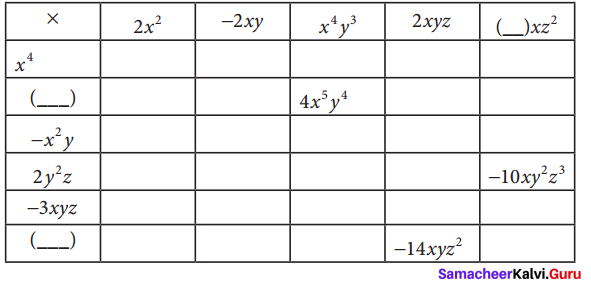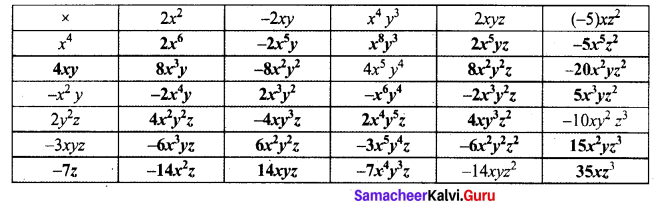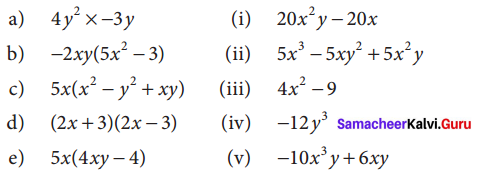Students can Download Maths Chapter 3 Algebra Ex 3.1 Questions and Answers, Notes Pdf, Samacheer Kalvi 8th Maths Book Solutions Guide Pdf helps you to revise the complete Tamilnadu State Board New Syllabus and score more marks in your examinations.

## Tamilnadu Samacheer Kalvi 8th Maths Solutions Term 1 Chapter 3 Algebra Ex 3.1

8th Maths Algebra Exercise 3.1 Question 1.
Multiply a monomial by a monomial.
(i) 6x, 4
(ii) -3x, 7y
(iii) -2m2, (-5m)3
(iv) a3, – 4a2b
(v) 2p2q3, -9pq2
Solution:
(i) 6x × 4 = (6 × 4) (x) = 24x
(ii) -3x × 7y = (-3 × 7) (x × y) = -21xy
(iii) (-2m2) × (-5m)3 = -2m2 × (-)3 (53 (m)3) = -2m2 × (-125m3)
= (-) × (-)(2 × 125)(m2 × m3) = + 250m5 = 250 m
(iv) a3 × (-4a2 b) = (-4) × (a3 × a2) × (b) = -4a5b
(v) (2p2q3) × (-9pq2) = (+) × (-) × (2 × 9) (p2 × p(q3 × q2)) = -18p3q5

Maths 8th Samacheer Kalvi Question 2.
Complete the tableSolution:8th Standard Maths 3.1 Solution Question 3.
Find the product of the terms.
(i) -2mn, (2m)2, -3mn
(ii) 3x2y, -3xy3, x2y2
Solution:
(i) (-2mn) × (2m)2 × (-3mn) = (-2mn) × 22 m2 × (-3mn) = (-2mn) × 4m2 × (-3mn)
= (-) (+)(-) (2 × 4 × 3) (m × m2 × m) (n × n)
= + 24 m4 n2

(ii) (32y) × (-31xy3) × (x2y2) = (+) × (-) × (+) × (3 × 3 × 1) (x2 × x × x2) x (y × y3 × y2)
= -9x5y6

Samacheer Kalvi Guru 8th Maths Question 4.
If l = 4pq2, b = -3p 2q, h = 2p3q3 then, find the value of 1 × b × h.
Solution:
Given l = 4pq2
b = -3p2q
h = 2p3q3
l × b × h = (4pq2) × (-3p2 q) × (2p3q3)
= (+) (-) (+) (4 × 3 × 2) (p × p2 × p3) (q2 × q × q3)
= -24p6q6

Samacheer Kalvi.Guru 8th Maths Question 5.
Expand
(i) 5x (2y – 3)
(ii) -2p (5p2 – 3p + 7)
(iii) 3mn (m3n3 – 5m2n + 7mn2)
(iv) x2 (x + y + z) y2 (x + y + z) + z2 (x – y – z)
Solution:
(i) 5x(2y – 3) = (5x) (2y) – (5x) (3)
= (5 × 2) (x × y) – (5 × 3) x
= 10xy – 15x

(ii) -2p (5p2 – 3p + 7) = (-2p) (5p2) + (-2p) (-3p) + (-2p) (7)
= [(-) (+) (2 × 5) (p × p2)] + [(-) (+) (2 × 3) (p × q)] + (-) (+) (2 × 7) p
= -10p3 + 6p2 – 14p

(iii) 3mn(m3n3 – 5m2n + 7mn2)
= (3mn) (m3n3) + (3mn) (-5m2n) + (3mn)(7mn2)
= (3) (m × m3) (n × n3) + (+) (-) (3 × 5) (m × m2) (n × n) + (3 × 7) (m × m)(n × n2)
= 3m4n4 – 15m3 n2 + 21m2n3

(iv) x2 (x + y + z) + y2 (x + y + z) + z2 (x – y – z)
= (x2 × x) + (x2 × y) + (x2 × z) + (y2 × x) + (y2 × y) + (y2 × z) + (z2 × x) + z2 (-y) + z2 (-z)
= x3 + x2y + x2z + xy2 + y3 + y2z + xz2 – yz2 – z3
= x3 + y3 – z2 + x2y + x2z + xy2 + zy2 + xz2 – yz2

Samacheer Kalvi Guru Maths 8th Question 6.
Find the product of
(i) (2x + 3)(2x – 4)
(ii) (y2 – 4) (2y2 + 3y)
(iii) (m2 – m) (5m2n2 – n2)
(iv) 3(x – 5) × 2(x – 1)
Solution:
(2x) (2x – 4) + 3 (2x – 4) = (2x) (2x – 4) + 3 (2x – 4)
= (2x × 2x) – 4 (2x) + 3(2x) – 3 (4)
= 4x2 – 8x + 6x – 12
= 4x2 + (- 8 + 6)x – 12
= 4x2 – 2x – 12

(ii) (y2 -4) (2y2 + 3y) = y2 (2y2 + 3y) – 4 (2y2 + 37)
= y2(2y2) + y2(3y) – 4(2y2) -4 (3y)
= 2y4 + 3y3 – 8y2 – 12y

(iii) (m2 – n) (5m2n2 – n2) = m2 (5m2n2 – n2) – n (5m2n2 – n2)
= m2 (5m2n2) + m2 (-n2) – n (5m2n2) + (-) (-) n (n2)
= 5m4n2 – m2n2 – 5m2n3 + n3

(iv) 3(x – 5) × 2(x – 1) = (3 × 2) (x – 5) (x – 1)
= 6 × [x (x – 1) – 5 (x- 1)]
= 6 [x.x – x . 1 – 5.x + (-1) (-) 5 1]
= 6 [x2 – x – 5x + 5] = 6 [x2 + (-1 – 5)x + 5]
= 6 [x2 – 6x + 5] = 6x2 – 36x + 30

Samacheer Kalvi 8 Maths Question 7.
Find the missing term.
(i) 6xy – × ______ = -12x3y
(ii) ________ × (-15m2n3p) = 45m3n3p2
(iii) 2y(5x2y – ___ + 3 ___) = 10x2y2 – 2xy + 6y3
Solution:
(i) 6xy – × (-2x2) = -12x3y
(ii) -3mp × (-15m2n3p) = 45m3n3p2
(iii) 2y(5x2y – x + 3 y2) = 10x2y2 – 2xy + 6y3

Samacheer Kalvi 8th Maths Book Solutions Question 8.
Match the following(A) iv, v, ii, i, iii
(B) v, iv, iii, ii, i
(C) iv, v, ii, iii, i
(D) iv, v, ii, iii, i
Solution:
(a) iv
(b) v
(c) ii
(d) iii
(e) i

Samacheer Kalvi 8 Maths Book Question 9.
A car moves at a uniform speed of (x + 30) km/hr. Find the distance covered by the car in (y + 2)hours. (Hint: distance = speed × time).
Solution:
Sppeed of the car = (x + 30) km / hr.
Time = (y + 2) hours
Distance = Speed × time = (x + 30) (y + 2) = x(y + 2) + 30 (y + 2) = x (y + 2) + 30 (y + 2)
= (x) (y) + (x) (2) + (30) (y) + (30) (2)
= xy + 2x + 30y + 60
Distance covered = (xy + 2x + 30y + 60) km

Objective Type Questions

Samacheer Kalvi Class 8 Maths Solutions Question 10.
The product of 7p3 and (2p2)2 is
(A) 14p2
(B) 28p7
(C) 9p7
(D) 11p12
Solution:
(B) 28p7

Samacheerkalvi.Guru 8th Maths Question 11.
The missing terms in the product -3m3 n × 9(- -) = ____ m4n3 are
(A) mn2, 27
(B) m2n, 27
(C) m2n2, -27
(D) mn2, -27
Solution
(A) mn2 ,27

Samacheer Kalvi Guru 8th Maths Guide Question 12.
If the area of a square is 36x4y2 then, its side is ______ .
(A) 6x4y2
(B) 8x2y2
(C) 6x2y
(D) -6x2y
Solution:
(C) 6x2y

Samacheer Kalvi 8th Maths Question 13.
If the area of a rectangle is 48m2n3 and whose length is 8mn2 then, its breadth is ____ .
(A) 6 mn
(B) 8m2n
(C) 7m2n2
(D) 6m2n2
Solution:
(A) 6mn

Maths Class 8 Samacheer Kalvi Question 14.
If the area of a rectangular land is (a2 – b2) sq.units whose breadth is (a – b) then, its length is _____
(A) a – b
(B) a + b
(C) a2 – b
(D) (a + b)2
Solution:
(B) a + b